[pdf] [ps.gz]
flame

# Results of flame propagation simulations with RFG method and cell-centered convection scheme

Andrei Smirnov
West Virginia University

All the results and visualizations presented here are done with the stand-alone software developed at WVU.

## Introduction

The effect of turbulence on flame propagation may be due to intersections of wrinkled flame surfaces and engulfment of unburnt regions inside the burnt regions. This effect can be qualitatively checked using computer simulations of a turbulent flow-field by spectral method and computing a simplified combustion process in this flow. In this work we use the RFG procedure and a newly developed convection/diffusion solver to test this effect.

## Turbulence model

The solution for a turbulent flow is given by the following equations (Kraichnan, 1970):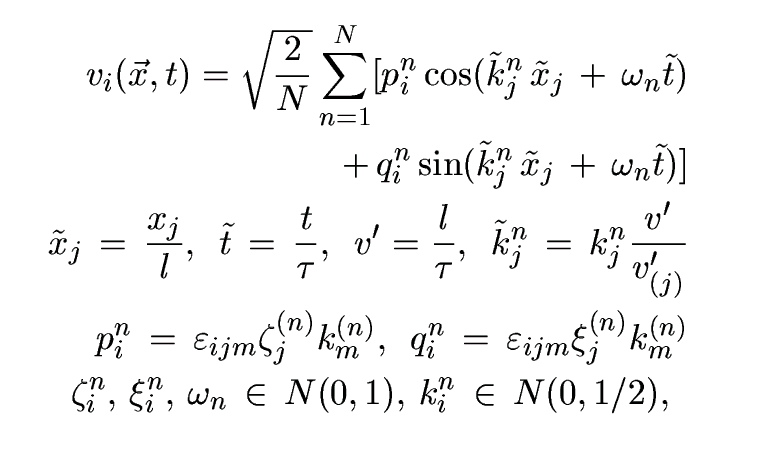where ,\tau are the length and time-scales of turbulence, \varepsilonijk is the permutation tensor used in vector product operation, and N(M,\sigma) is a normal distribution with mean M and standard deviation \sigma. Numbers kjn, \omegan represent a sample of n wavenumber vectors and frequencies of the modeled turbulence spectrum

 E(k)=16(((2)/(\pi)))1/2k4\exp(-2k2) (5)

A way of generating random flow-field with this method, which represents a simplified spectral technique, is covered more in detail in (Get a pdf-copy of the paper).

## Combustion model

A convection/diffusion solver was written to solve for the transport of a scalar variable in a turbulent flow field generated by the RFG procedure above. A scalar variable used was allowed to change between 0.0 and 1.0 and corresponds to a progress variable P of a premixed combustion reaction. For a qualitative test of the effect of flame-front acceleration due to turbulent velocity fluctuations a simple combustion model was used, where the progress variable in each cell was updated every time-step according to the following criteria:

IF (P>R) P=1.0

where R is an ignition limit that was set to 0.5 in this qualitative study. In the absence of turbulence (quiescent flow field) the flame propagation velocity was determined entirely by the diffusion rate constant D and in the absence of diffusion, i.e. D=0, the flame front propagation velocity was 0. When the turbulent velocity perturbation were introduced the flame front started propagating, engulfing the unburnt region.

## Results

Figures below show the results of preliminary simulations of flame propagation in a modeled Gaussian turbulent field computed on a 16x16x16 cube. Since an unstructured, tetrahedral grid is used the number of cells in that grid is actually greater than the number of nodes, i.e. Nnodes=16*16*16=4096, Ncells=5*16*16*16=20480. That many cells are shown in the figures, each cell represented by a single colored point. Red color corresponds to fully burnt, blue - to fully unburnt state, colors in between correspond to partially burnt state.

### Non-dimensional problem parameters:

• Physical parameters
• 
Correlations of turbulent velocity fluctuations:

uu=1.0
uv=0.0
vv=1.0
uw=0.0
vw=0.0
ww=1.0

Turbulent time scale: 1.0

Turbulent length scale: 1.0

Initial distribution of P along the X-axis:

P=1.0 for 0<X<4
P=0.0 for 4<X<16


• Computational parameters
• 
Grid dimensions: 16 x 16 x 16
Spectral sample size: N=1000
Time-step: 0.05



where the spectral sample size parameter N refers to the equation above.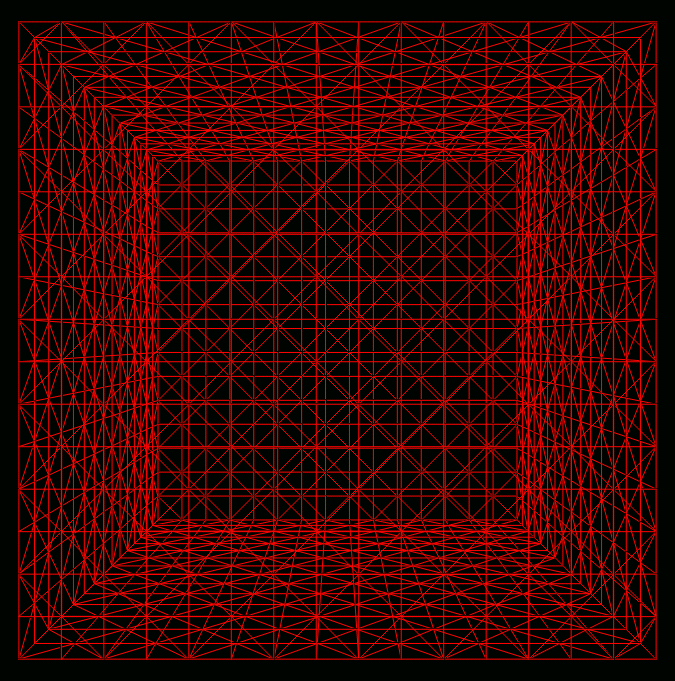163 cube grid used in simulations.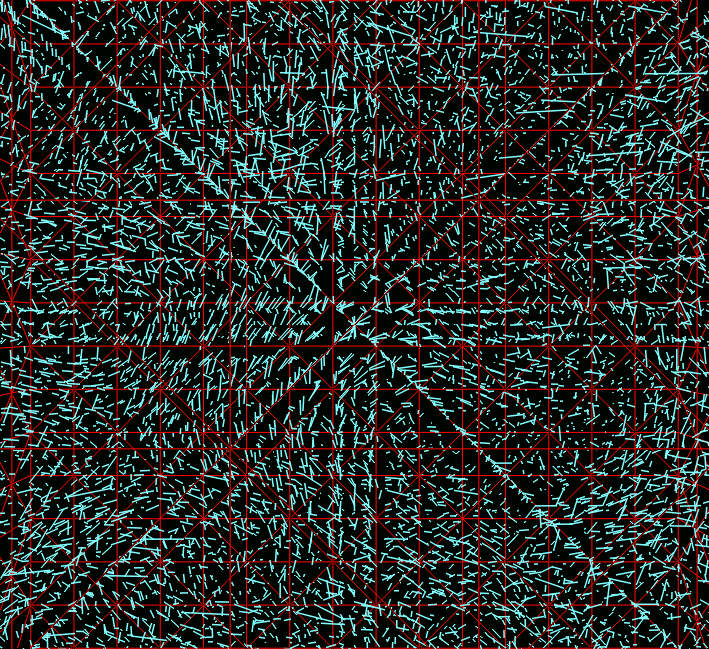Animation of transient veolocity field (mpg)
Snapshot of turbulent velocity field generated by RFG.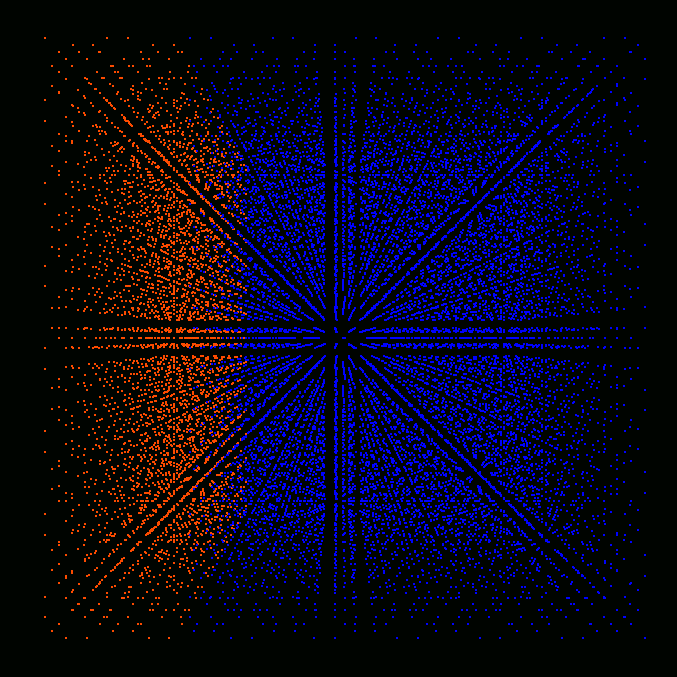Initial distribution of progress variable (concentration ratio of burnt/unburnt species).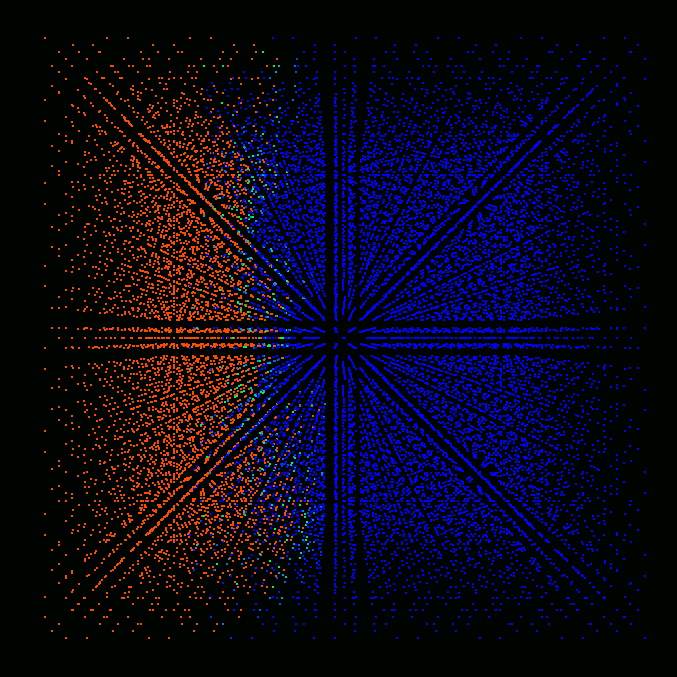Time = 1.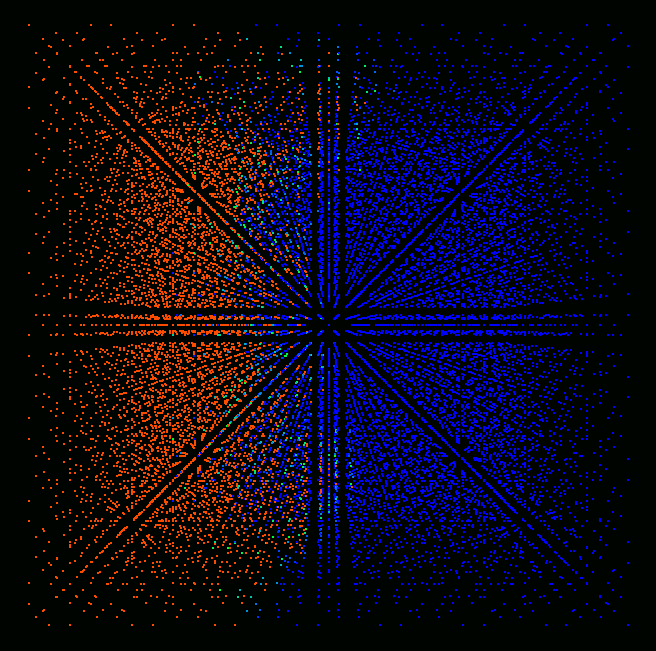Time = 2.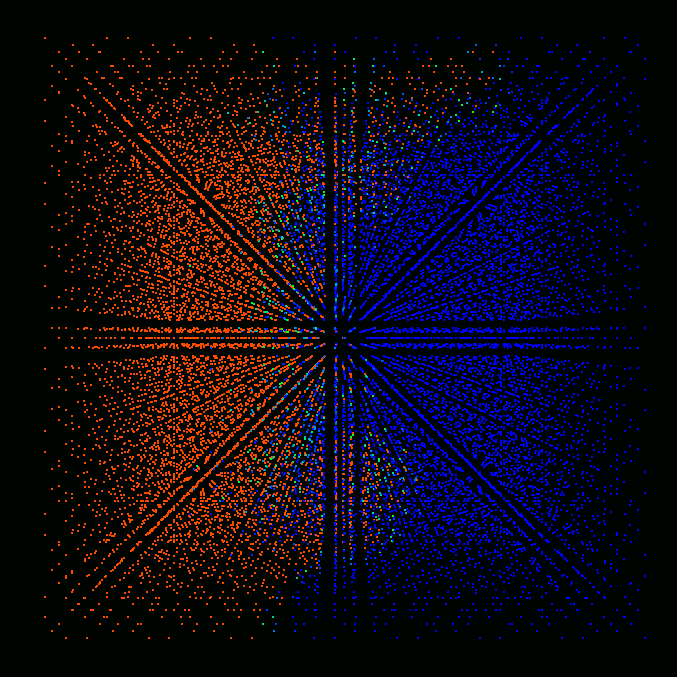Time = 3.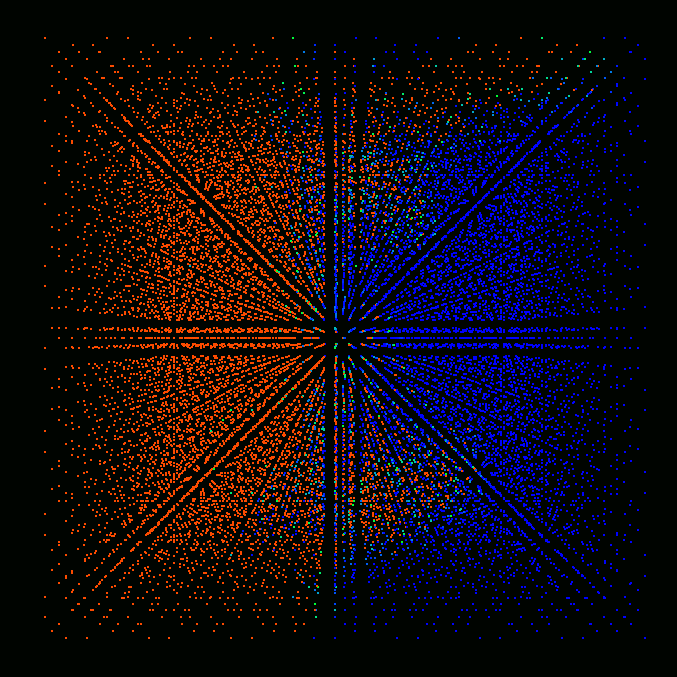Time = 4.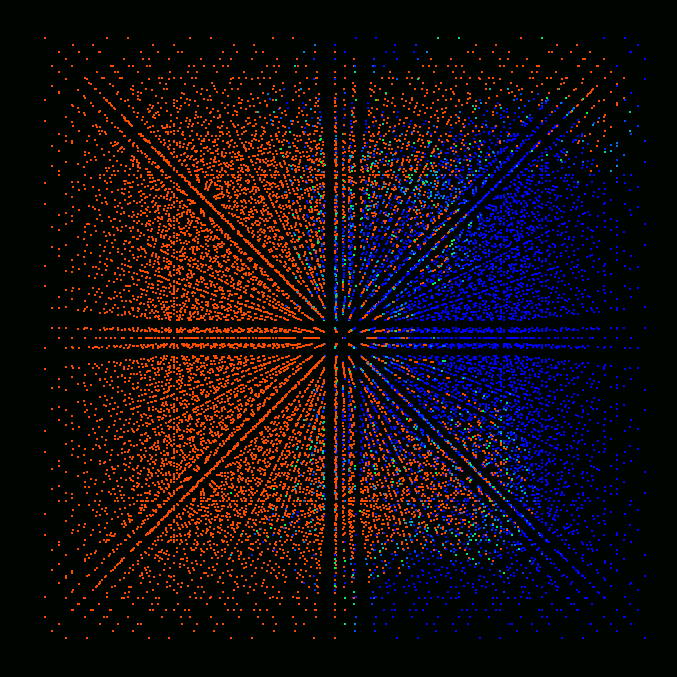Time = 5.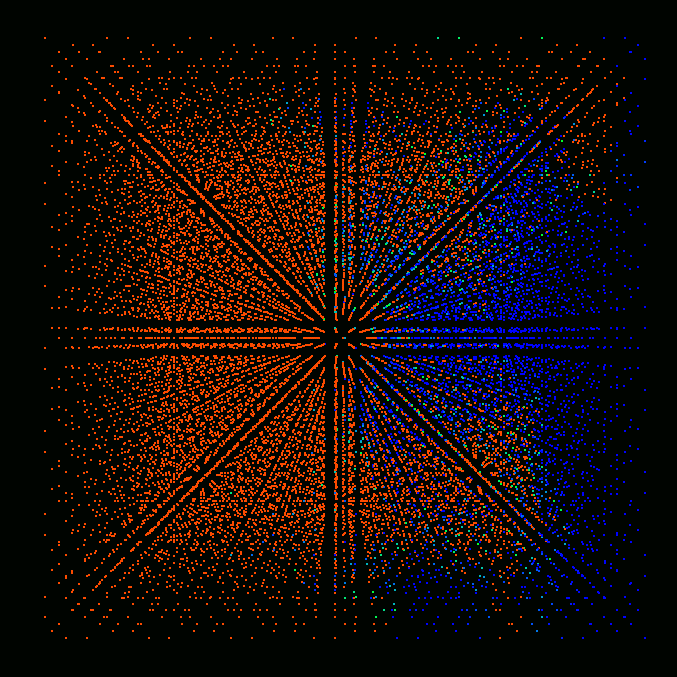Time = 6.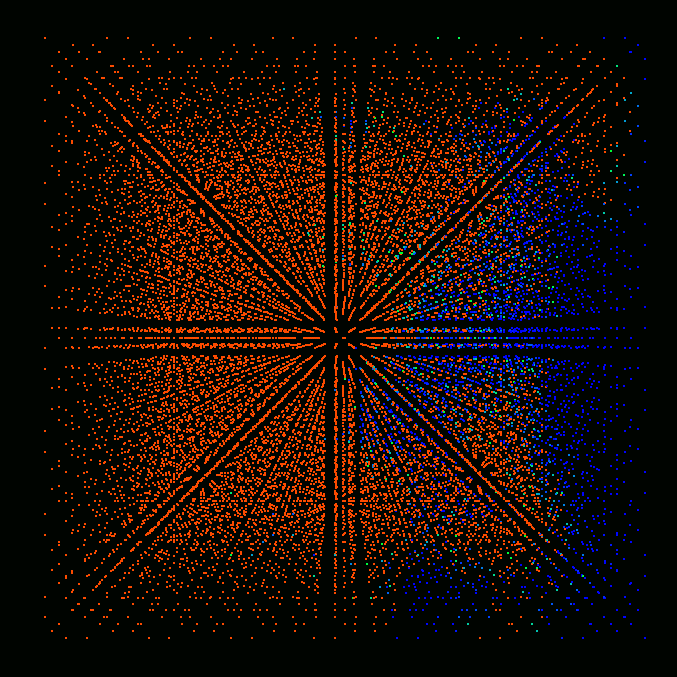Time = 7.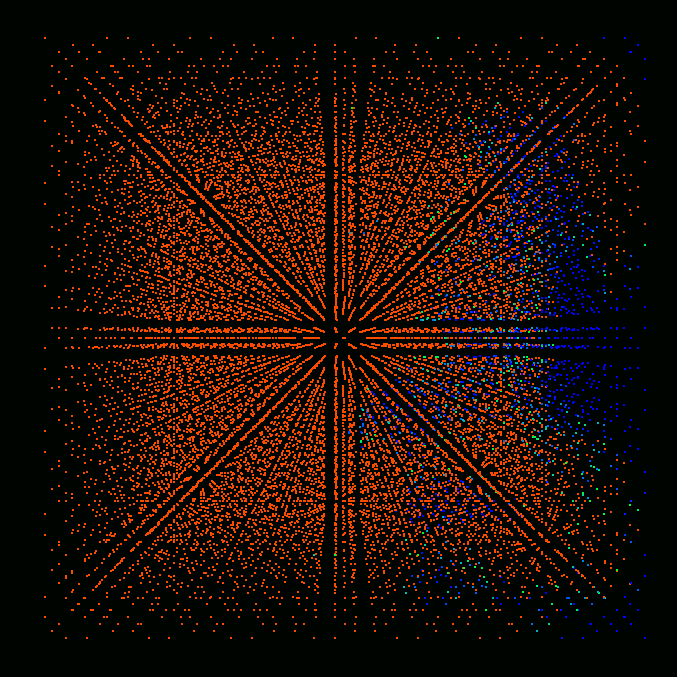Time = 8.

## Conclusions

The results show the increase of flame propagation speed from 0 to approximately 1 due to turbulent fluctuations. Formation of unburnt and partially burnt pockets inside the burnt region is also evident from the figures.

## Acknowledgements

I would like to thank Dr. Valeri Golovitchev from Chalmers University for the initial ideas and the inspiration needed for this work.

The results were presented at The International Conference "Micromixing 2004" (ICMM-2004).

Abstract [pdf] [ps.gz]